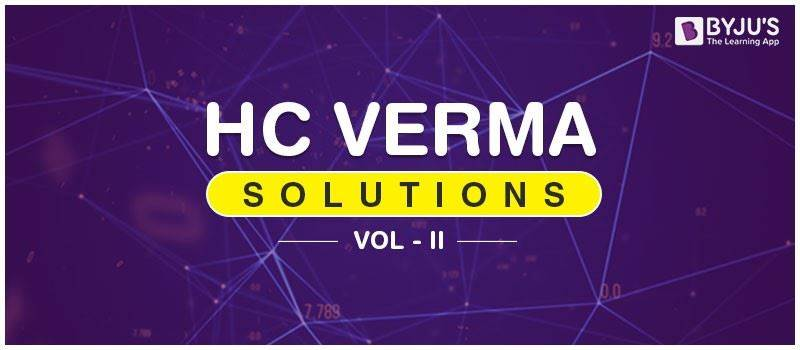# HC Verma Solutions Vol 2HC Verma Solutions vol 2 comprises of Physics topics covered in the class 12th syllabus. These solutions are extremely helpful for students preparing for medical and engineering entrance exams like JEE, NEET, BITSAT, etc. HC Verma vol 2 solutions for all the chapters are provided here. These solutions are prepared by our subject experts to present the factual information to the students related to problems covered in HC Verma book. It provides an in-depth knowledge of the topics covered in class 12 physics syllabus of JEE Main and Advanced as well. The solutions cover concepts like Heat, Calorimetry, Thermodynamics, Heat Transfer, Electric Potential, Gauss’s Law, Capacitors, Electric Current, Magnetic Fields, Permanent Magnets, Magnetic Properties, Electromagnetic Induction, Alternating Current, Electric Current, Photoelectric Effect, Bohr’s Theory, X-rays, Semiconductors, Nucleus, and Theory of Relativity.

## Highlights Of HC Verma Vol 2 Solutions

The solutions provided here offers answers to all the questions, tests and exercises. Thus students are suggested to practice these solutions regularly to enhance problem-solving capabilities and developing strong analytical and reasoning skills.

• The solutions for HC Verma will help students in understanding the highly complicated problems discussed in the physics textbook.
• Also, the solutions will give an idea of the type of questions that students can expect in the competitive exams. Students can also access all the solutions based on the complex concepts of physics to improve self-evaluation skills. You will be able to download chapter-wise HC Verma volume 2 solutions from below.

## HC Verma Vol 2 Physics Solutions

Access free HC Verma Solutions pdf for vol 2 from the given table.

 Vol 2 Chapter 1 – Heat and Temperature Vol 2 Chapter 2 – Kinetic Theory of Gases Vol 2 Chapter 3 – Calorimetry Vol 2 Chapter 4 – Laws of Thermodynamics Vol 2 Chapter 5 – Specific Heat Capacities of gases Vol 2 Chapter 6 – Heat Transfer Vol 2 Chapter 7 – Electric Field and Potential Vol 2 Chapter 8 – Gauss’s Law Vol 2 Chapter 9 – Capacitor Vol 2 Chapter 10 – Electric Current in Conductors Vol 2 Chapter 11 – Electric Current Vol 2 Chapter 12 – Magnetic Field Vol 2 Chapter 13 – Magnetic Field Due to Current Vol 2 Chapter 14 – Permanent Magnets Vol 2 Chapter 15 – Magnetic Properties of Matter Vol 2 Chapter 16 – Electromagnetic Induction Vol 2 Chapter 17 – Alternating Current Vol 2 Chapter 18 – Electromagnetic Waves Vol 2 Chapter 19 – Electric Current Through Gases Vol 2 Chapter 20 – Photoelectric Effect Vol 2 Chapter 21 – Bohr’s Theory and Physics of Atom Vol 2 Chapter 22 – X-Rays Vol 2 Chapter 23 – Semiconductor and Semiconductor Devices Vol 2 Chapter 24 – The Nucleus Vol 2 Chapter 25 – The Special Theory of Relativity

To access more subject-related content for IIT JEE and NEET preparation along with important questions, sample papers, topic-wise mock tests, question papers keep exploring BYJU’S website.